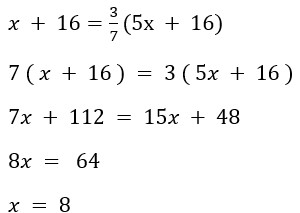# Problems on ages

## Problems on ages

Problem on ages is a common topic on which questions in the quantitative aptitude section of all Government exams are based.

Although the questions in this Problems on ages section may appear to be difficult, if a candidate grasps the concept, he or she will be able to simply answer them.

In this problems on ages article, candidates can find the formulas, example questions, etc.

In this post, we’ll go through the many types of questions on Age Based problem that appear on various government exams, as well as some tips and tactics that can help you answer the questions more quickly and efficiently.

Candidates can also look at some basic formulas for answering Problems on ages questions.

Problems on ages Important Formulas

• If you are assuming the current age to be x, then the age after n years will be (x+n) years.
• If you are assuming the current age to be x, then the age before n years will be (x-n) years.
• If the age is given in the form of a ratio, for example, p:q, then the age shall be considered as qx and px
• If you are assuming the current age to be x, then n times the current age will be (x×n) years
• If you are assuming the current age to be x, then 1/n of the age shall be equal to (x/n) years

#### Let’s see some problems on Ages

Questions 1. A mother is twice as old as her son. If 20 years ago, the age of the mother was 10 times the age of the son, what is the present age of the mother?

Answer.  Let the age of son = x
Therefore, mother’s age = 2x
20 Years ago mother’s age = 2x – 20
20 Years ago son’s age = x – 20
According to Question
2x – 20 = 10 ( x – 20 )
2x – 20 = 10x – 200
8x = 180
x = 180/8 = 22.5
So, the age of mother = 2x = 2(22.5) = 45 years

Question 2. The ratio of the ages of Neeraj and Divya is 9 : 10 respectively. Ten years ago, the ratio of their ages was 4 : 5 respectively. What is the present age of Divya ?

Answer.  Let Neeraj’s age = 9x and Divya’s age = 10x
10 years age, Neeraj’s age = 9x – 10, Divya’s age = 10x – 10Divya’s present age = 10x = 10.2 = 20 years

Question 3. Ram’s age after 6 years will be three–sevenths of her father’s age. 10 years ago, the ratio of their ages was 1 : 5. What is Ram’s father’s age at present?

Answer. Let’s Ram’s age 10 years ago is x
Ram’s father age 10 years ago = 5x
Ram’s age after 6 years = x + 10 + 6 = x + 16
Ram’s father age after 6 years = 5x + 10 + 6 = 5x + 16

According to QuestionsRam’s father present age = 5x + 10 = 5×8 + 10 = 50 Years

#### Attempt Quantitative Aptitude Topic Wise Online Test Series

Recommended PDF’s for :

#### Most important PDF’s for Bank, SSC, Railway and Other Government Exam : Download PDF Now

AATMA-NIRBHAR Series- Static GK/Awareness Practice Ebook PDF Get PDF here
The Banking Awareness 500 MCQs E-book| Bilingual (Hindi + English) Get PDF here
AATMA-NIRBHAR Series- Banking Awareness Practice Ebook PDF Get PDF here
Computer Awareness Capsule 2.O Get PDF here
AATMA-NIRBHAR Series Quantitative Aptitude Topic-Wise PDF Get PDF here
AATMA-NIRBHAR Series Reasoning Topic-Wise PDF Get PDF Here
Memory Based Puzzle E-book | 2016-19 Exams Covered Get PDF here
Caselet Data Interpretation 200 Questions Get PDF here
Puzzle & Seating Arrangement E-Book for BANK PO MAINS (Vol-1) Get PDF here
ARITHMETIC DATA INTERPRETATION 2.O E-book Get PDF here
3